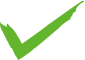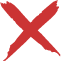# GPA Calculator

This GPA calculator calculates your grade point average using a 4.33 or 4.0 scale. The GPA Scales can be found here: 4.33 GPA Scale and 4.0 GPA Scale.

Input Type:

GPA Scale:Hint -- If your school does not use credits, simply input "1" in the "credits" section for each course.

Note -- The conversion scales used in this GPA calculator are accepted by most institutions. However, if there is a scale that you would like to see added, let us know in the comments section below!

12345678910